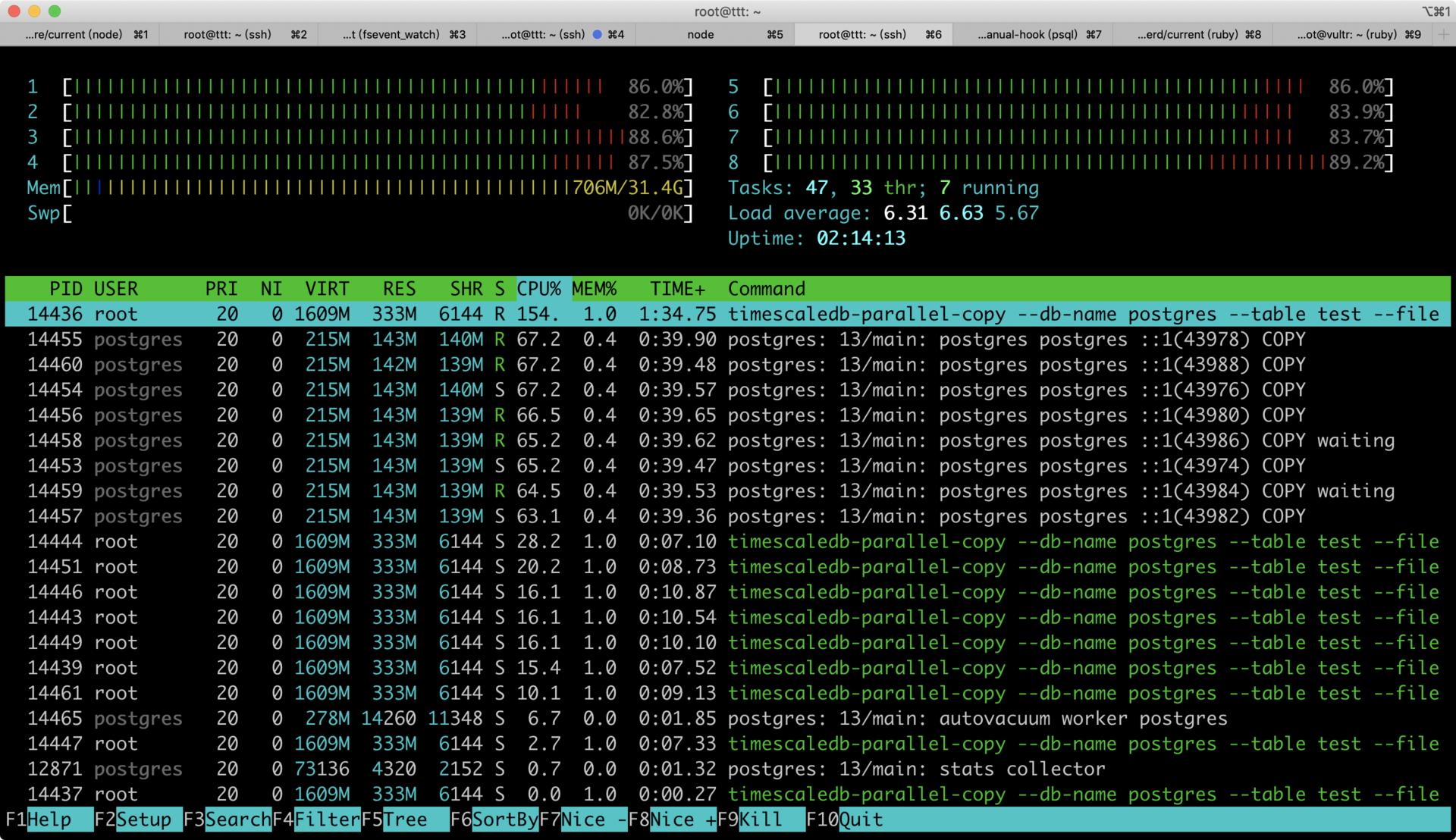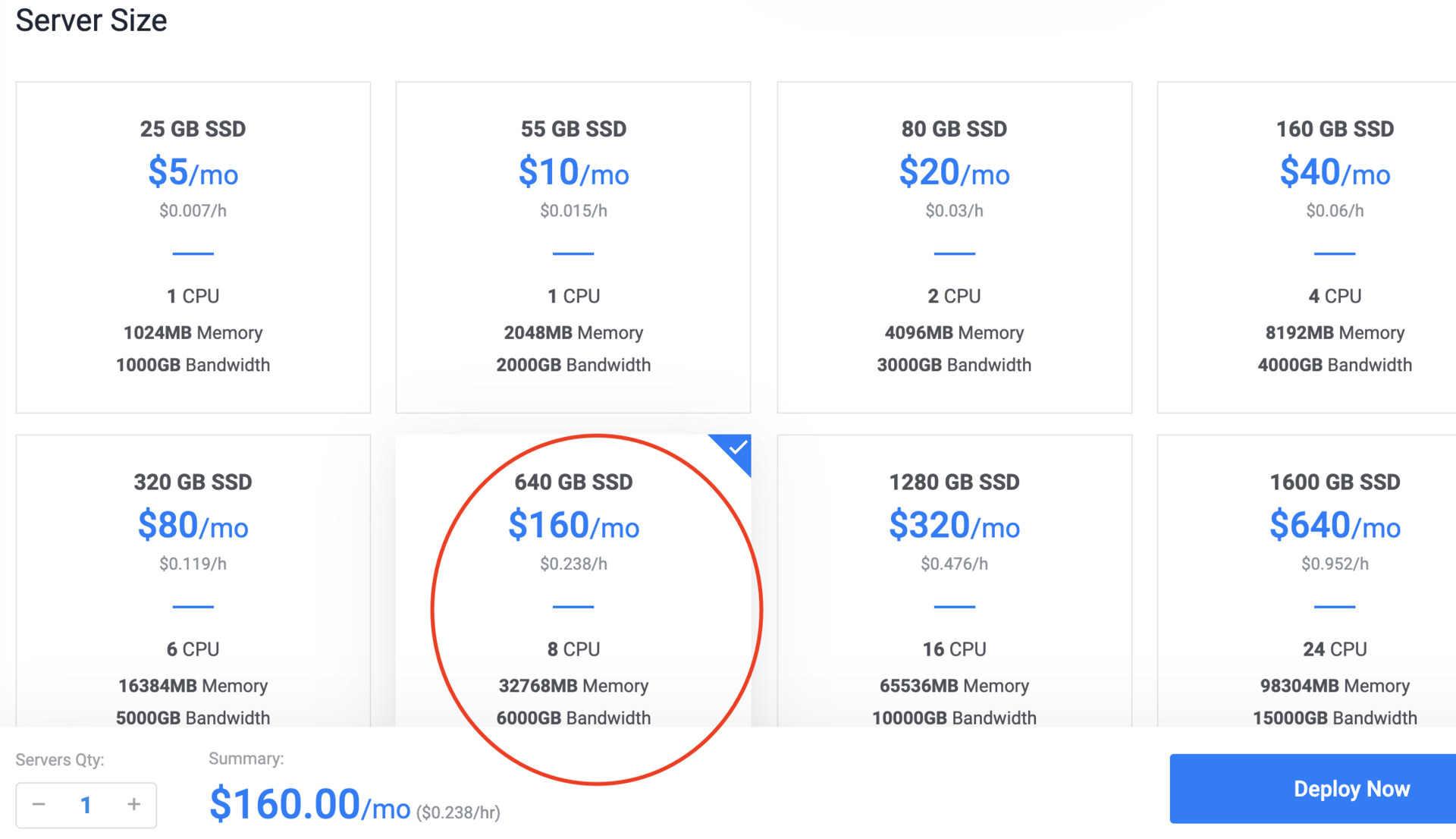# 数据库 如何 10 分钟内插入 13 亿条记录？

hooopo · 2020年10月10日 · 最后由 ny 回复于 2020年10月10日 · 1436 次阅读

``````f = File.open("ii.csv", "w")
(1..1280000000).each do |x|
f.puts [x, Random.random_number(1000000000), "#{x}.com"].join(",")
end
f.close
``````

csv 概览：

``````tail -fn20 ii.csv
1279999981,379768240,1279999981.com
1279999982,440776589,1279999982.com
1279999983,194045965,1279999983.com
1279999984,643339201,1279999984.com
1279999985,397295532,1279999985.com
1279999986,308045177,1279999986.com
1279999987,860093304,1279999987.com
1279999988,557636470,1279999988.com
1279999989,882497774,1279999989.com
1279999990,987416658,1279999990.com
1279999991,728315013,1279999991.com
1279999992,163951092,1279999992.com
1279999993,524652,1279999993.com
1279999994,871673632,1279999994.com
1279999995,833545894,1279999995.com
1279999996,635775438,1279999996.com
1279999997,19686670,1279999997.com
1279999998,243310061,1279999998.com
1279999999,706814112,1279999999.com
1280000000,701386384,1280000000.comv
``````

``````timescaledb-parallel-copy --db-name postgres --table test --file ./ii.csv --workers 8 --reporting-period 30s -connection "host=localhost user=postgres password=helloworld sslmode=disable" -truncate -batch-size 100000
````````````timescaledb-parallel-copy --db-name postgres --table test --file ./ii.csv --workers 8 --reporting-period 30s -connection "host=localhost user=postgres password=helloworld sslmode=disable" -truncate -batch-size 100000
at 30s, row rate 2049993.13/sec (period), row rate 2049993.13/sec (overall), 6.150000E+07 total rows
at 1m0s, row rate 1913075.25/sec (period), row rate 1981529.69/sec (overall), 1.189000E+08 total rows
at 1m30s, row rate 1930261.28/sec (period), row rate 1964442.55/sec (overall), 1.768000E+08 total rows
at 2m0s, row rate 1943332.35/sec (period), row rate 1959165.00/sec (overall), 2.351000E+08 total rows
at 2m30s, row rate 1966667.61/sec (period), row rate 1960665.52/sec (overall), 2.941000E+08 total rows
at 3m0s, row rate 1919998.29/sec (period), row rate 1953887.65/sec (overall), 3.517000E+08 total rows
at 3m30s, row rate 1950001.96/sec (period), row rate 1953332.55/sec (overall), 4.102000E+08 total rows
at 4m0s, row rate 1949999.02/sec (period), row rate 1952915.86/sec (overall), 4.687000E+08 total rows
at 4m30s, row rate 1929999.19/sec (period), row rate 1950369.56/sec (overall), 5.266000E+08 total rows
at 5m0s, row rate 1873333.69/sec (period), row rate 1942665.98/sec (overall), 5.828000E+08 total rows
at 5m30s, row rate 1913172.63/sec (period), row rate 1939984.56/sec (overall), 6.402000E+08 total rows
at 6m0s, row rate 1843488.31/sec (period), row rate 1931943.89/sec (overall), 6.955000E+08 total rows
at 6m30s, row rate 1816666.49/sec (period), row rate 1923076.40/sec (overall), 7.500000E+08 total rows
at 7m0s, row rate 1962938.14/sec (period), row rate 1925924.19/sec (overall), 8.089000E+08 total rows
at 7m30s, row rate 1950394.13/sec (period), row rate 1927555.19/sec (overall), 8.674000E+08 total rows
at 8m0s, row rate 1963333.08/sec (period), row rate 1929791.31/sec (overall), 9.263000E+08 total rows
at 8m30s, row rate 1963333.49/sec (period), row rate 1931764.38/sec (overall), 9.852000E+08 total rows
at 9m0s, row rate 1966666.04/sec (period), row rate 1933703.36/sec (overall), 1.044200E+09 total rows
at 9m30s, row rate 1963333.35/sec (period), row rate 1935262.83/sec (overall), 1.103100E+09 total rows
at 10m0s, row rate 1949999.36/sec (period), row rate 1935999.66/sec (overall), 1.161600E+09 total rows
at 10m30s, row rate 1966667.45/sec (period), row rate 1937460.03/sec (overall), 1.220600E+09 total rows
at 11m0s, row rate 1933333.38/sec (period), row rate 1937272.46/sec (overall), 1.278600E+09 total rows
COPY 1280000000
``````